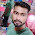### Program 171:To Print value of Cosine Mathematically and using Library Function

Program 171:

```#include<stdio.h>
#include<math.h>
int factorial(int);
main()
{
//cos x = 1 - x 2 / 2! + x 4 / 4! - x 6 / 6! + ...
int accuracy,count=0,k=0;
float x,temp,cosine=0;

printf("Enter value x(in degrees) to find cos x value\n");
scanf("%f",&x);
temp=x;
x=(float)x*(3.14159/180);
printf("Enter value to get more accurate\n 3 for least and more for more accuracy\n");
scanf("%d",&accuracy);

do
{
if(count%2==0)
{
cosine=(float)cosine+(pow(x,k)/factorial(k));
}
if(count%2==1)
{
cosine=(float)cosine-(pow(x,k)/factorial(k));
}
count++;
k=k+2;
}while(count<=accuracy);

printf("cos(%f)=%f calcualted mathematically\n",temp,cosine);
printf("cos(%f)=%f calculated using library function\n",temp,cos(x));
}

int factorial(int val)
{
int factorial=1,i;
for(i=1;i<=val;i++)
{
factorial=factorial*i;
}
return(factorial);

}

```
Explanation:

The Explanation is same as the program 170 to print sine value except the formula is changed to
`cos x = 1 - x 2 / 2! + x 4 / 4! - x 6 / 6! + ...`
`Here k is initialized to 0 for getting even number powers to base etc `

Output:

#### 1 comment:

1.college-party-guide One of the most important rituals for students in college is to throw the most memorable student dorm celebration.
factorial hundred In the last few days, the “factorial of 100” is one of the top subjects and a lot of maths geeks compute it using voice assistants such as Alexa, Shiri, etc.
factorial hundred In the last few days, the “factorial of 100” is one of the top subjects and a lot of maths geeks compute it using voice assistants such as Alexa, Shiri, etc.
gigolo The term”gigolo” usually refers to the person who lives an arranged lifestyle that includes many of these relationships in a series, rather than having any other support system.